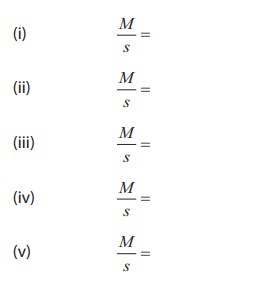Home | | Physics 11th std | Non - Uniform Bending - Verification of Relation Between Load and Depression Using Pin and Microscope

# Non - Uniform Bending - Verification of Relation Between Load and Depression Using Pin and Microscope

To verify the relation between the load and depression using non-uniform bending of a beam.

NON â€“ UNIFORM BENDING â€“ VERIFICATION OF RELATION BETWEEN LOAD AND DEPRESSION USING PIN AND MICROSCOPE

## AIM

To verify the relation between the load and depression using non-uniform bending of a beam.

## APPARATUS REQUIRED

A long uniform beam (usually a metre scale), two knife â€“ edge supports, mass hanger, slotted masses, pin, vernier microscope

## FORMULA

M/s = a constant

where

M â†’ Load applied (mass) (kg)

s â†’ depression for the applied load(metre)

## DIAGRAM## PROCEDURE

Â·           Place the two knife â€“ edges on the table.

Â·           Place the uniform beam (metre scale) on top of the knife edges.

Â·           Suspend the mass hanger at the centre. A pin is attached at the centre of the scale where the hanger is hung.

Â·           Place a vernier microscope in front of this arrangement

Â·           Adjust the microscope to get a clear view of the pin

Â·         Make the horizontal cross-wire on the microscope to coincide with the tip of the pin. (Here mass hanger is the dead load M).

Â·         Note the vertical scale reading of the vernier microscope

Â·         Add the slotted masses one by one in steps of 0.05 kg (50 g) and take down the readings.

## OBSERVATIONS

To find M/s## MODEL GRAPH

A graph between M and s can be drawn by taking M along X- axis and s along Y â€“ axis.

This is a straight line.## CALCULATION## RESULT

Â·         The ratio between mass and depression for each load is calculated. This is found to be constant.

Â·           Thus the relation between load and depression is verified by the method of non-uniform bending of a beam.

Tags : Physics Laboratory Practical Experiment , 11th Physics : Practical Experiment
Study Material, Lecturing Notes, Assignment, Reference, Wiki description explanation, brief detail
11th Physics : Practical Experiment : Non - Uniform Bending - Verification of Relation Between Load and Depression Using Pin and Microscope | Physics Laboratory Practical Experiment